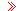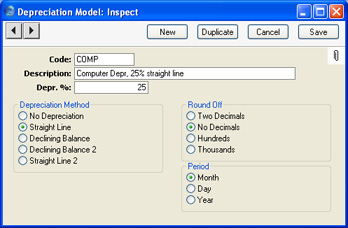Language:

Version 8.4hansaworld.comFAQForums

HansaManualsIntroduction to Settings in the Assets ModuleAccount Usage AssetsAsset Display GroupsAsset CategoriesAsset N/L ClassesCustomersDepartmentsDepreciation ModelsInflation CoefficientsNumber Series - Asset StatusNumber Series - DisposalsNumber Series - RevaluationsNumber Series - Revaluation FactorsRevaluation FactorsUniversityTutorials for Standard Accounts

Depreciation Models

Depreciation Models are the formulae that you will use to calculate the depreciation of your Assets. You can use up to two Models in each Asset.

To enter a Depreciation Model, open the 'Settings' list in the Assets module and double-click 'Depreciation Models'. The 'Depreciation Models: Browse' window is opened, showing Depreciation Models already entered. Click [New] in the Button Bar or use the Ctrl-N (Windows and Linux) or ⌘-N (Macintosh) keyboard shortcut. The 'Depreciation Model: New' window is opened.Code
Enter a unique code by which the Model can be identified. You can use a maximum of five characters.

Name
The complete name of the model.

%
Enter the percentage by which annual depreciation is to be calculated. This will be applied to the Purchase Value of the Asset (entered on the 'Purchase' card of the Asset record) or its Fiscal Value, or to the Starting Value in the latest Revaluation record, if one exists.

Depreciation Method
Each Depreciation Model can use one of four depreciation methods. The method determines how the depreciation is calculated. Check with local legislation as to which method is appropriate for your country of operation.
No Depreciation
No depreciation is calculated.

Straight Line
This method means that the Asset is written down by the same amount each year. It uses the Purchase Value or Fiscal Value as the basis for the calculation, unless the Asset has been revalued. If so, the Starting Value in the latest Revaluation will be the basis for the calculation.

For example, if an Asset cost 100,000 and is to be depreciated by 20% p.a. using the Straight Line method, it will depreciate by 20,000 each year and will be written off after 5 years.

Declining Balance
This method causes the base value of the Asset to decline by the depreciation amount each year.

For example, an Asset which cost 100,000 which is to be depreciated by 20% p.a. using the Declining Balance method will depreciate by 20,000 in the first year and by 16,000 in the second year (20% of 100,000-20,000) and so on. Such an Asset will never be completely written off. In some countries such as Portugal there may be special rules about writing off such an Asset at the end of its useful life. Please refer to your local Hansa representative and your auditor for advice.

If you are calculating depreciation monthly, the base value of the Asset will decline each month. In the above example, depreciation in the first month will be 100,000 x 20% x 1/12 = 1666.66. In the second month, the depreciation will be (100,000 - 1666.66) x 20% x 1/12 = 1638.89, in the third month it will be (100,000 - 1666.66 - 1638.89) x 20% x 1/12 = 1611.57, and so on through the year.

Declining Balance 2
This method is identical to Declining Balance described above if you are calculating depreciation annually.

If you are calculating depreciation monthly, this method is half-way between Straight Line and Declining Balance. It uses the Straight Line method to calculate figures for each month in a calendar year, but the base value is reduced for the next year on a Declining Balance basis. For example, depreciation in the first month of an Asset which cost 100,000 and which is to be depreciated by 20% p.a. using this method will be 100,000 x 20% x 1/12 = 1666.66. In the second month, the depreciation will again be 1666.66, and so on. Every January, the base value will be re-calculated (to 100,000 - (12 x 1666.66) = 80,000 in the example, assuming it was purchased the previous January). Monthly depreciation in the next year will be 80,000 x 20% x 1/12 = 1333.33. The recalculation of the base value always takes place in January, irrespective of when the Asset was purchased, although the result will depend on the purchase date. For example, if the sample Asset was purchased in July, the base value will change to 100,000 - (6 x 1666.66) = 90,000 the following January.

Depending on advice from your auditor or other financial adviser, this may be the preferred Declining Balance method for use in the UK.

Straight Line 2
This method is used in Lithuania and is identical to the Straight Line method described above if you are calculating depreciation for Assets for which there are no Revaluations.

If there is a Revaluation, then depreciation will be calculated using this formula:

(Value on date of Revaluation + Change in Value) * Depreciation %

For example, an Asset was purchased for 2400 on 31/12/2003. Its Residual Value is 1. It will be depreciated by 25% p.a. using this method. To start with, depreciation is identical to that calculated using the Straight Line method, so the value on 31/12/2005 is 2400 - (600 x 2) = 1200.

The Asset is revalued on 1/1/2006. The Start. Value in the Revaluation is 3000. So, for 2006, depreciation will be (1200 + (3000 - 2400)) x 25% = 450.00, and it will be fully depreciated on 31/12/2009.

If you were using the Straight Line method, annual depreciation after the Revaluation would be 3000 x 25% = 750.00.
Period
These options are relevant to Straight Line Depreciation Models only. Declining Balance Models always use the Month option. The option that you choose here will only be used in the Asset History - Portugal report, the Depreciations Report, the three Portuguese Fiscal reports and the Subsidy Assets report. In all other circumstances, you will choose a period at the moment of calculating depreciation or producing a report.
Month
This option will divide the yearly depreciation percentage by 12 to obtain a monthly percentage. For example, an Asset worth 50,000 being depreciated by 5% p.a. will be depreciated by 208.33 per month (50,000 x 0.05 / 12). This figure will always be the same, irrespective of the number of days in the month. The minimum period for the depreciation calculation in reports will be one month, starting from the first day of the month.

Day
This option will divide the yearly depreciation percentage by 365 and multiply it by the number of days in the month to obtain a monthly percentage. For example, an Asset worth 50,000 being depreciated by 5% p.a. will be depreciated by 212.33 per 31 day month (50,000 x 0.05 / 365 x 31). This figure will change, depending on the number of days in the month. The number of days is calculated from the depreciation period entered in the specification window of the report or Maintenance function. There is no minimum period for the depreciation calculation.

Year
If you use this option, the minimum period for the depreciation calculation will be one year, starting from the first day of the calendar year.
Round Off
Use these options to determine the rounding method to be used in any depreciation calculations that use this Model.